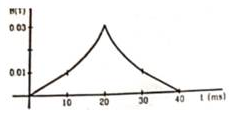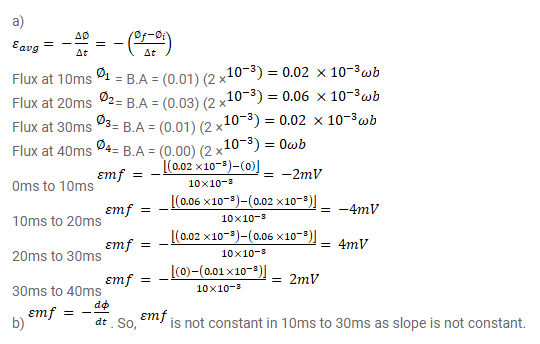# (a) The magnetic field in a region varies as shown in the given figure.

Question:

(a) The magnetic field in a region varies as shown in the given figure. Calculate the average induced emf in a conducting loop of area $2.0 \times 10^{-3} \mathrm{~m}^{2}$ placed perpendicular to the field in each of the $10 \mathrm{~ms}$ intervals shown.

(b) In which intervals is the emf not constant? Neglect the behaviour near the ends of $10 \mathrm{~ms}$ intervals.Solution: